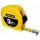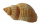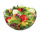#### Number of problems found: 512 and 1 8th plus 1 and 1 3rd =What is 4 1/2+2/7-213/14?Why does 1 3/4 + 2 9/10 equal 4.65? How do you solve this?3 3/4 + 2 3/5 + 5 1/2 Show your solution.
• Pizza fractionsAnn ate a third of a pizza and then another quater. Total part of pizza eaten by Ann and how much pizza is left?Add two mixed fractions: 2 4/6 + 1 3/6Add this two mixed numbers: 1 5/6 + 2 2/11=
• Series and sequencesFind a fraction equivalent to the recurring decimal? 0.435643564356
• Conversion of unitsComplete the following length data
• FractionFraction ? write as fraction a/b, a, b is integers numerator/denominator.
• Decimal to fractionWrite decimal number 8.638333333 as a fraction A/B in the basic form. Given decimal has infinite repeating figures.7 is added to the sum of 4/5 and 6/7
• Sum of fractionsWhat is the sum of 2/3+3/5?
• Roses and tulipsAt the florist are 50 tulips and 5 times less roses. How many flowers are in flower shop?
• Length subtractingExpress in mm: 5 3/10 cm - 2/5 mmDivide number 135 into two additions so that one adds 30 more than 2/5 of the other add. Write the bigger one.
• James 2James collected 24 sea shells on the seashore to be shared with his 2 friends. What fractional part of the seashells will each one get if it is distributed evenly? ​We need two tenths kg of a carrot, one tenth of peas and three tenths of of tomatoes to make salad. Express the fraction of the weight of the vegetables to be salad. Convert the result to grams.
• BarrelsPeter has 42 barrels. One-sixth of them are filled with lemonade, one third is filled with wine and half is empty. Calculate how many barrels are with each filling.
• Two numbersThe sum of two numbers is 1. Identify this two numbers if you know that the half of first is equal to the third of second number.

Do you have an interesting mathematical word problem that you can't solve it? Submit a math problem, and we can try to solve it.

We will send a solution to your e-mail address. Solved examples are also published here. Please enter the e-mail correctly and check whether you don't have a full mailbox.

Please do not submit problems from current active competitions such as Mathematical Olympiad, correspondence seminars etc...

Need help calculate sum, simplify or multiply fractions? Try our fraction calculator. Adding Problems. Fraction Word Problems.